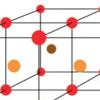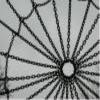# Resources tagged with: Generalising

Filter by: Content type:
Age range:
Challenge level:

### There are 148 results

Broad Topics > Thinking Mathematically > Generalising### 2001 Spatial Oddity

##### Age 11 to 14 Challenge Level:

With one cut a piece of card 16 cm by 9 cm can be made into two pieces which can be rearranged to form a square 12 cm by 12 cm. Explain how this can be done.### Tilted Squares

##### Age 11 to 14 Challenge Level:

It's easy to work out the areas of most squares that we meet, but what if they were tilted?### Tourism

##### Age 11 to 14 Challenge Level:

If you can copy a network without lifting your pen off the paper and without drawing any line twice, then it is traversable. Decide which of these diagrams are traversable.##### Age 11 to 14 Challenge Level:

A little bit of algebra explains this 'magic'. Ask a friend to pick 3 consecutive numbers and to tell you a multiple of 3. Then ask them to add the four numbers and multiply by 67, and to tell you. . . .### Shear Magic

##### Age 11 to 14 Challenge Level:

Explore the area of families of parallelograms and triangles. Can you find rules to work out the areas?### Dotty Triangles

##### Age 11 to 14 Challenge Level:

Imagine an infinitely large sheet of square dotty paper on which you can draw triangles of any size you wish (providing each vertex is on a dot). What areas is it/is it not possible to draw?### Go Forth and Generalise

##### Age 11 to 14

Spotting patterns can be an important first step - explaining why it is appropriate to generalise is the next step, and often the most interesting and important.### Frogs

##### Age 11 to 14 Challenge Level:

How many moves does it take to swap over some red and blue frogs? Do you have a method?### Handshakes

##### Age 11 to 14 Challenge Level:

Can you find an efficient method to work out how many handshakes there would be if hundreds of people met?### Christmas Chocolates

##### Age 11 to 14 Challenge Level:

How could Penny, Tom and Matthew work out how many chocolates there are in different sized boxes?### Picturing Square Numbers

##### Age 11 to 14 Challenge Level:

Square numbers can be represented as the sum of consecutive odd numbers. What is the sum of 1 + 3 + ..... + 149 + 151 + 153?### Picturing Triangular Numbers

##### Age 11 to 14 Challenge Level:

Triangular numbers can be represented by a triangular array of squares. What do you notice about the sum of identical triangle numbers?### Games Related to Nim

##### Age 5 to 16

This article for teachers describes several games, found on the site, all of which have a related structure that can be used to develop the skills of strategic planning.### Nim-like Games

##### Age 7 to 16 Challenge Level:

A collection of games on the NIM theme### Sum Equals Product

##### Age 11 to 14 Challenge Level:

The sum of the numbers 4 and 1 [1/3] is the same as the product of 4 and 1 [1/3]; that is to say 4 + 1 [1/3] = 4 � 1 [1/3]. What other numbers have the sum equal to the product and can this be so. . . .### Magic Letters

##### Age 11 to 14 Challenge Level:

Charlie has made a Magic V. Can you use his example to make some more? And how about Magic Ls, Ns and Ws?### Enclosing Squares

##### Age 11 to 14 Challenge Level:

Can you find sets of sloping lines that enclose a square?##### Age 11 to 14 Challenge Level:

List any 3 numbers. It is always possible to find a subset of adjacent numbers that add up to a multiple of 3. Can you explain why and prove it?### Finding 3D Stacks

##### Age 7 to 11 Challenge Level:

Can you find a way of counting the spheres in these arrangements?### Steps to the Podium

##### Age 7 to 14 Challenge Level:

It starts quite simple but great opportunities for number discoveries and patterns!### Hidden Rectangles

##### Age 11 to 14 Challenge Level:

Rectangles are considered different if they vary in size or have different locations. How many different rectangles can be drawn on a chessboard?### Squares, Squares and More Squares

##### Age 11 to 14 Challenge Level:

Can you dissect a square into: 4, 7, 10, 13... other squares? 6, 9, 12, 15... other squares? 8, 11, 14... other squares?### Taking Steps

##### Age 7 to 11 Challenge Level:

In each of the pictures the invitation is for you to: Count what you see. Identify how you think the pattern would continue.### Special Sums and Products

##### Age 11 to 14 Challenge Level:

Find some examples of pairs of numbers such that their sum is a factor of their product. eg. 4 + 12 = 16 and 4 × 12 = 48 and 16 is a factor of 48.### Winning Lines

##### Age 7 to 16

An article for teachers and pupils that encourages you to look at the mathematical properties of similar games.### Searching for Mean(ing)

##### Age 11 to 16 Challenge Level:

If you have a large supply of 3kg and 8kg weights, how many of each would you need for the average (mean) of the weights to be 6kg?### More Number Pyramids

##### Age 11 to 14 Challenge Level:

When number pyramids have a sequence on the bottom layer, some interesting patterns emerge...### Cuboid Challenge

##### Age 11 to 16 Challenge Level:

What's the largest volume of box you can make from a square of paper?### Circles, Circles

##### Age 5 to 11 Challenge Level:

Here are some arrangements of circles. How many circles would I need to make the next size up for each? Can you create your own arrangement and investigate the number of circles it needs?### Sliding Puzzle

##### Age 11 to 16 Challenge Level:

The aim of the game is to slide the green square from the top right hand corner to the bottom left hand corner in the least number of moves.### Keep it Simple

##### Age 11 to 14 Challenge Level:

Can all unit fractions be written as the sum of two unit fractions?### Partitioning Revisited

##### Age 11 to 14 Challenge Level:

We can show that (x + 1)² = x² + 2x + 1 by considering the area of an (x + 1) by (x + 1) square. Show in a similar way that (x + 2)² = x² + 4x + 4### Egyptian Fractions

##### Age 11 to 14 Challenge Level:

The Egyptians expressed all fractions as the sum of different unit fractions. Here is a chance to explore how they could have written different fractions.### Cubes Within Cubes Revisited

##### Age 11 to 14 Challenge Level:

Imagine starting with one yellow cube and covering it all over with a single layer of red cubes, and then covering that cube with a layer of blue cubes. How many red and blue cubes would you need?### Beach Huts

##### Age 11 to 14 Challenge Level:

Can you figure out how sequences of beach huts are generated?### Number Pyramids

##### Age 11 to 14 Challenge Level:

Try entering different sets of numbers in the number pyramids. How does the total at the top change?### Arithmagons

##### Age 11 to 16 Challenge Level:

Can you find the values at the vertices when you know the values on the edges?### More Twisting and Turning

##### Age 11 to 16 Challenge Level:

It would be nice to have a strategy for disentangling any tangled ropes...### Seven Squares - Group-worthy Task

##### Age 11 to 14 Challenge Level:

Choose a couple of the sequences. Try to picture how to make the next, and the next, and the next... Can you describe your reasoning?### Sums of Pairs

##### Age 11 to 16 Challenge Level:

Jo has three numbers which she adds together in pairs. When she does this she has three different totals: 11, 17 and 22 What are the three numbers Jo had to start with?”### How Much Can We Spend?

##### Age 11 to 14 Challenge Level:

A country has decided to have just two different coins, 3z and 5z coins. Which totals can be made? Is there a largest total that cannot be made? How do you know?### Got It

##### Age 7 to 14 Challenge Level:

A game for two people, or play online. Given a target number, say 23, and a range of numbers to choose from, say 1-4, players take it in turns to add to the running total to hit their target.### Konigsberg Plus

##### Age 11 to 14 Challenge Level:

Euler discussed whether or not it was possible to stroll around Koenigsberg crossing each of its seven bridges exactly once. Experiment with different numbers of islands and bridges.### One, Three, Five, Seven

##### Age 11 to 16 Challenge Level:

A game for 2 players. Set out 16 counters in rows of 1,3,5 and 7. Players take turns to remove any number of counters from a row. The player left with the last counter looses.### Make 37

##### Age 7 to 14 Challenge Level:

Four bags contain a large number of 1s, 3s, 5s and 7s. Pick any ten numbers from the bags above so that their total is 37.##### Age 11 to 16

Dave Hewitt suggests that there might be more to mathematics than looking at numerical results, finding patterns and generalising.### Nim-7

##### Age 5 to 14 Challenge Level:

Can you work out how to win this game of Nim? Does it matter if you go first or second?### ...on the Wall

##### Age 11 to 14 Challenge Level:

Explore the effect of reflecting in two intersecting mirror lines.### Who Is the Fairest of Them All ?

##### Age 11 to 14 Challenge Level:

Explore the effect of combining enlargements.### Squares in Rectangles

##### Age 11 to 14 Challenge Level:

A 2 by 3 rectangle contains 8 squares and a 3 by 4 rectangle contains 20 squares. What size rectangle(s) contain(s) exactly 100 squares? Can you find them all?